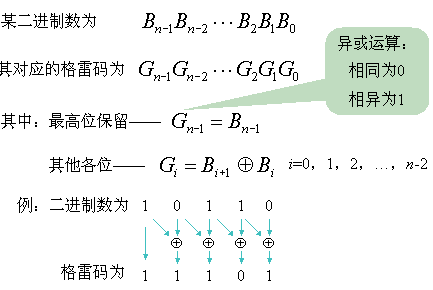# 异步fifo的设计(FPGA)

FIFO的本质是RAM， 先进先出

fifo位宽（每个数据的位宽）
FIFO有同步异步两种，同步即读写时钟相同，异步即读写时钟不相同

1、读空信号如何产生？写满信号如何产生？

当读指针赶上写指针的时候，写指针等于读指针意味着最后一个数据被读完，此时读空信号有效

7 --> 8 ，格雷码从 0100 --> 1100 ,只有最高位发生变化其余位相同
6 --> 9  , 格雷码从 0101 --> 1101 , 只有最高位发生变化其余位相同

7的格雷码与8的格雷码的最高位不同，其余位相同，所以判断出为写满。这就出现误判了，同样套用在 6 和 9，5 和 10等也会出现误判。图 1

2、由于是异步FIFO的设计，读写时钟不一样，在产生读空信号和写满信号时，会涉及到跨时钟域的问题，如何解决？

（1）将写时钟域的写指针同步到读时钟域，将同步后的写指针与读时钟域的读指针进行比较产生读空信号
（2）将读时钟域的读指针同步到写时钟域，将同步后的读指针与写时钟域的写指针进行比较产生写满信号

我们如果直接用二进制编码的读写指针去完成上述的两种同步是不行的，使用格雷码更合适，为什么呢？

3、由于设计的时候读写指针用了至少两级寄存器同步，同步会消耗至少两个时钟周期，势必会使得判断空或满有所延迟，这会不会导致设计出错呢？

4、多位二进制码如何转化为格雷码wclk  wrst_n  winc wdata //写时钟、写复位、写请求、写数据     这几个与写有关的全部与wclk同步
rclk  rrst_n    rinc   rdata //读时钟、读 复位、读 请求、读 数据    这几个与读有关的全部与rclk同步
wfull                              //写满  与wclk同步
rempty                          // 读空 与rclk同步

1、fifo.v  是顶层模块，作用是将各个小模块例化联系起来module fifo
#(
parameter DSIZE = 8,
parameter ASIZE = 4
)
(
output [DSIZE-1:0] rdata,
output             wfull,
output             rempty,
input  [DSIZE-1:0] wdata,
input              winc, wclk, wrst_n,
input              rinc, rclk, rrst_n
);

wire   [ASIZE:0]   wptr, rptr, wq2_rptr, rq2_wptr;
// synchronize the read pointer into the write-clock domain
sync_r2w  sync_r2w
(
.wq2_rptr    (wq2_rptr),
.rptr        (rptr    ),
.wclk        (wclk    ),
.wrst_n      (wrst_n  )
);

// synchronize the write pointer into the read-clock domain
sync_w2r  sync_w2r
(
.rq2_wptr(rq2_wptr),
.wptr(wptr),
.rclk(rclk),
.rrst_n(rrst_n)
);

//this is the FIFO memory buffer that is accessed by both the write and read clock domains.
//This buffer is most likely an instantiated, synchronous dual-port RAM.
//Other memory styles can be adapted to function as the FIFO buffer.
fifomem
#(DSIZE, ASIZE)
fifomem
(
.rdata(rdata),
.wdata(wdata),
.wclken(winc),
.wfull(wfull),
.wclk(wclk)
);

//this module is completely synchronous to the read-clock domain and contains the FIFO read pointer and empty-flag logic.
rptr_empty
#(ASIZE)
rptr_empty
(
.rempty(rempty),
.rptr(rptr),
.rq2_wptr(rq2_wptr),
.rinc(rinc),
.rclk(rclk),
.rrst_n(rrst_n)
);

//this module is completely synchronous to the write-clock domain and contains the FIFO write pointer and full-flag logic
wptr_full
#(ASIZE)
wptr_full
(
.wfull(wfull),
.wptr(wptr),
.wq2_rptr(wq2_rptr),
.winc(winc),
.wclk(wclk),
.wrst_n(wrst_n)
);
endmodule
View Code

2、fifomem.v  生成存储实体，FIFO 的本质是RAM,因此在设计存储实体的时候有两种方法：用数组存储数据或者调用RAM的IP核module fifomem
#(
parameter  DATASIZE = 8, // Memory data word width
parameter  ADDRSIZE = 4  // 深度为8即地址为3位即可，这里多定义一位的原因是用来判断是空还是满，详细在后文讲到
) // Number of mem address bits
(
output [DATASIZE-1:0] rdata,
input  [DATASIZE-1:0] wdata,
input                 wclken, wfull, wclk
);

ifdef RAM   //可以调用一个RAM IP核
// instantiation of a vendor's dual-port RAM
my_ram  mem
(
.dout(rdata),
.din(wdata),
.wclken(wclken),
.wclken_n(wfull),
.clk(wclk)
);
else  //用数组生成存储体
// RTL Verilog memory model
localparam DEPTH = 1<<ADDRSIZE;   // 左移相当于乘法，2^4
reg [DATASIZE-1:0] mem [0:DEPTH-1]; //生成2^4个位宽位8的数组
always @(posedge wclk)  //当写使能有效且还未写满的时候将数据写入存储实体中，注意这里是与wclk同步的
if (wclken && !wfull)
endif
endmodule
View Code

3、sync_r2w.v 将 rclk 时钟域的格雷码形式的读指针同步到 wclk 时钟域，简单来讲就是用两级寄存器同步，即打两拍module sync_r2w
#(
)
(
input                   wclk, wrst_n
);

always @(posedge wclk or negedge wrst_n)
if (!wrst_n) begin
wq1_rptr <= 0;
wq2_rptr <= 0;
end
else begin
wq1_rptr<= rptr;
wq2_rptr<=wq1_rptr;
end
endmodule
View Code

4、sync_w2r.v 将 wclk 时钟域的格雷码形式的写指针同步到 rclk 时钟域module sync_w2r
(
input                   rclk, rrst_n
);

always @(posedge rclk or negedge rrst_n)
if (!rrst_n)begin
rq1_wptr <= 0;
rq2_wptr <= 0;
end
else begin
rq1_wpt <= wptr;
rq2_wptr <= rq1_wptr;
end

endmodule
View Code

5、rptr_empty.v 将 sync_w2r.v 同步后的写指针与 rclk 时钟域的读指针进行比较生成都空信号module rptr_empty
#(
)
(
output reg                rempty,
output reg [ADDRSIZE  :0] rptr,  //格雷码形式的读指针
input                     rinc, rclk, rrst_n
);
// GRAYSTYLE2 pointer
//将二进制的读指针与格雷码进制的读指针同步
always @(posedge rclk or negedge rrst_n)
if (!rrst_n) begin
rbin <= 0;
rptr <= 0;
end
else begin
rbin<=rbinnext; //直接作为存储实体的地址
rptr<=rgraynext;//输出到 sync_r2w.v模块，被同步到 wrclk 时钟域
end
assign rbinnext  = rbin + (rinc & ~rempty); //不空且有读请求的时候读指针加1
assign rgraynext = (rbinnext>>1) ^ rbinnext; //将二进制的读指针转为格雷码
// FIFO empty when the next rptr == synchronized wptr or on reset
assign rempty_val = (rgraynext == rq2_wptr); //当读指针等于同步后的写指针，则为空。
always @(posedge rclk or negedge rrst_n)
if (!rrst_n)
rempty <= 1'b1;
else
rempty <= rempty_val;

endmodule
View Code

6、wptr_full.v 将  sync_r2w.v 同步后的读指针与wclk 时钟域的写指针进行比较生成写满信号module wptr_full
#(
)
(
output reg                wfull,
input                     winc, wclk, wrst_n
);
// GRAYSTYLE2 pointer
always @(posedge wclk or negedge wrst_n)
if (!wrst_n)
{wbin, wptr} <= 0;
else
{wbin, wptr} <= {wbinnext, wgraynext};
assign wbinnext  = wbin + (winc & ~wfull);
assign wgraynext = (wbinnext>>1) ^ wbinnext; //二进制转为格雷码
//-----------------------------------------------------------------
always @(posedge wclk or negedge wrst_n)
if (!wrst_n)
wfull  <= 1'b0;
else
wfull  <= wfull_val;

endmodule
View Code

7、测试文件timescale 1ns /1ns

module test();
reg  [7:0] wdata;
reg           winc, wclk, wrst_n;
reg           rinc, rclk, rrst_n;
wire [7:0] rdata;
wire           wfull;
wire          rempty;

fifo

u_fifo (
.rdata(rdata),
.wfull(wfull),
.rempty(rempty),
.wdata (wdata),
.winc  (winc),
.wclk  (wclk),
.wrst_n(wrst_n),
.rinc(rinc),
.rclk(rclk),
.rrst_n(rrst_n)
);
localparam CYCLE = 20;
localparam CYCLE1 = 40;

//时钟周期，单位为ns，可在此修改时钟周期。

//生成本地时钟50M
initial begin
wclk = 0;
forever
#(CYCLE/2)
wclk=~wclk;
end
initial begin
rclk = 0;
forever
#(CYCLE1/2)
rclk=~rclk;
end

//产生复位信号
initial begin
wrst_n = 1;
#2;
wrst_n = 0;
#(CYCLE*3);
wrst_n = 1;
end

initial begin
rrst_n = 1;
#2;
rrst_n = 0;
#(CYCLE*3);
rrst_n = 1;
end

always  @(posedge wclk or negedge wrst_n)begin
if(wrst_n==1'b0)begin
winc <= 0;
rinc <= 0;
end
else begin
winc <= $random; rinc <=$random;
end
end

always  @(posedge rclk or negedge rrst_n)begin
if(rrst_n==1'b0)begin
rinc <= 0;
end
else begin
rinc <= $random; end end always@(*)begin if(winc == 1) wdata=$random ;
else
wdata = 0;
end
endmodule
View Code

8、仿真结果posted @ 2016-12-05 15:18  aslmer  阅读(42420)  评论(33编辑  收藏  举报